## Results

### Experiment-based computational modelling

Impact loading applied to the mussel is modelled as an instantaneous initial velocity of the mussel’s body, reflecting that it accounts for 99.5% of the total mass of the system. According to the experiment, the measured impact force of 17.8 N yields an instantaneous velocity of 2.0 m s−1 at the center of mass of the mussel where the byssus threads attach to (see the Methods section for details). We test the instantaneous velocity both in the vertical direction for the tension mode and along the substrate for the shearing mode, as shown in Fig. 5a,c, respectively. We find that the proximal part undergoes significant deformation as the mussel moves apart while the distal part remains in a relaxed state with zero tensile force. The tensile force in the proximal part is rather sensitive to the movement of the mussel from its equilibrated position as it reaches a peak within 0.01 s. However, the tensile force in the distal part at that moment is only halfway to reaching the peak force, as shown in Fig. 5b,d. The peak force in the distal part is much smaller than that of the proximal part, and reaches 80% in the tension mode and only 53% in the shearing mode. The proximal part connected to the mussel effectively buffers the impact to the adhesion plaque. Therefore, we can understand the role of the proximal part as a safety belt of the byssus network, as it is critical to absorb the impact loading. We increase the initial speed stepwise in the simulations, and find that the maximum impact speed for failure is 3.3 m s−1 for tension and 3.5 m s−1 for shearing. Those initial velocities correspond to a maximum impact force of 48.5 N, which is over nine times that of the static strength. This value agrees well with the highest impact forces on mussels that have been recorded at shores and reported in the literature2.

### Energy absorption and dynamical strength by structure

In order to understand the underlying mechanism, and specifically how the material distribution in the byssus thread (that is, the ratio of stiff material versus the entire thread, here denoted by C) is adapted to dynamic loading, we systematically alter the ratio between the distal and proximal parts and the geometry of the byssus network, and investigate how those characteristics affect the dynamic response of the byssus network under impact loading. The total energy of the system (the sum of kinetic energy and potential energy) as a function of time before the mussel reaches maximum deformation is shown in Fig. 6a,b for the tension and shearing modes, respectively. The energy dissipation for byssus threads with a proximal part is significantly higher than the byssus thread composed of a pure distal part (C=1). We measure the time when the total energy decreases to half of its initial value as shown in Fig. 6c,d, where C=1 corresponds to the slowest total energy dissipation while decreasing C corresponds to faster energy dissipation. The transition between C=0.5 and 1 contributes mostly to the increment of the rate of energy dissipation. We repeat the measurement of the decay time of the kinetic energy to its half value (Fig. 6c,d and Supplementary Fig. S4) and find that a larger C leads to a greater advantage in terms of its ability to decrease the kinetic energy. This shows that the combination of those two materials benefits the energy absorption of the byssus network as the distal part quickly transits the instant kinetic energy into potential energy, while the proximal part dissipates the kinetic energy by viscosity. For pure elasticity, the peak force is proportional to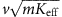where Keff is the effective stiffness of the network and m is the mass of the mussel while for pure damping the peak force is proportional to eff where ηeff is the effective coefficient of viscosity, which depends on the thread diameter and the value of water viscosity. The reaction force experienced by the mussel is proportional to (detailed derivation in the Methods section)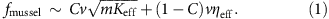## Discussion

The few and thin byssus threads that mussels use to affix themselves to the environment provides them with significant lack of restrictions for feeding and migration in order to cope with environmental changes. According to our results, direct impacts with speeds lower than 3.3 m s−1 (~12 km h−1) to the mussel are safe for the byssus network. Because of the affinity of the mussels to the substrate, the flow speed around the mussel never exceeds 10% of the free-stream velocity18,19. Thereby, the mussel can remain attached to the sea bottom or hulls of ships of up to 120 km h−1 surface wave speeds (or ship speeds), explaining how mussels survive and thrive in such extreme conditions.

The structural and mechanical lessons gained by investigating the byssus network could provide us with important design principles that can be applied in the fabrication of interfacial materials that can function effectively under dynamic loadings, such as attachments to ships, submarines, in wind turbines, or for applications in space technologies4,25,26. From a slightly different perspective, we have found that the use of about 20% soft material in mussel threads is crucial in light of impact absorption and force reduction for the entire animal. This result is reminiscent of the Pareto principle27 (also known as the 80/20 rule), given the fact that a tiny amount of causes account for almost all of the effects. This principle reflects the finding that the greatest force deduction comes from the soft material, which makes up only a small portion of the overall thread (Fig. 1b)1,15,16. This is a rule of thumb that has wide applications in social sciences, economics and business because it provides the empirical outcome of the balance between two competing mechanisms generally regarded as cost and gain. It is noted that this analogy is a conjecture at this point and that caution needs to be taken in the comparison. The agreement between the observation in mussel and the Pareto principle indicates that the design of byssus threads may reflect an optimal state of the competition between energy dissipation and thread deformation.

Nature features an interesting repertoire of building materials, selecting the appropriate material and structure for distinct purposes. Here we find that, the remarkable properties of mussel adhesion are not merely owing to the strength of the adhesion pads, but rather rely on a specific distribution of stiff and soft materials in a unique geometry. This concept identified here is derived from an attachment system but has implications beyond this specific application, and provides a general advance in the understanding of mitigation strategies for impact loading. Hence, it could be applied in the design of attachments and implants in bioengineering, structural engineering and architecture, bio-inspired material design and polymer science.

## Methods

### Mussel sample preparation and experimental setup

The samples of M. edulis were collected in the native environment of Boston harbour area together with substrates made of clay. The cage was kept under sea for 3 weeks to ensure the mussels’ attachment. We carefully cleaned the samples with sea water before further testing the adhesion between the mussel and the substrate. The tensile tests were carried out in room temperature with a standard tensile testing machine (MTS Corporation, Eden Prairie, MN, USA). In each of the tensile tests, the substrate was clamped to the cross head, which moved at a constant rate of 0.1 mm s−1 displacement. The attached mussel was held by a force transducer where the reaction force was measured. The cross head displacement is measured using a linear voltage displacement transducer mounted inside the actuator. The total force applied to the mussel and the total displacement of the substrate were scanned with 1 Hz and saved to the connected data collector. Each sample was kept in a sea water tank before performing the test.

We apply an initial instant speed to the mussel to model the result of impact loading. The relevant velocities are converted from the in situ force history measured at shores. The impact force of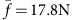is measured as the reference force at shore2, taking the force signal as general sinusoidal wave, the peak force equals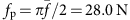. Considering the tethered Dacron string as pure elastic, the initial speed v0 can thus be estimated by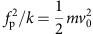, where m is the weight of the mussel model and k is the elastic constant of the Dacron string. The values of those parameters used in the in situ force measurement study are m=0.045 kg and k=9,600 N m−1 for the 130 lbs string (consider 30% extensibility, 20 cm in length)2. This string has been used to tether between the mussel and force sensor to carry out the in situ measurements2. Those parameters yield an initial velocity of 2 m s−1 of the mussel model. The initial speed of 3.5 m s−1 is the maximum for our model before it starts to rupture. According to fpv0 relationship, this maximum initial speed yields to an impact loading of fp=48.5 N. This value agrees well with the highest impact forces on mussels that is recorded and reported in the literature2.

### Computational model of the byssus thread

Each byssus thread is modelled by three segments: the proximal part, distal part and adhesion plaque (Fig. 1b). We use a simple bead-spring model to model the proximal and distal part with a diameter of d=250±40 μm as given by the experimental measurement (Supplementary Fig. S2). This result agrees with the earlier measurement of byssus thread diameter in literature (275 μm for M. californianus1). We use the tensile force-strain response of a single thread at different regions as measured experimentally in literature to define the nonlinear stiffness of the spring in tension1. Each of the stress–strain curves is composed of three regimes: elastic, yielding and post-yielding. We design the spring stiffness accordingly by using a multi-polynomial potential for the springs. The equilibrated spring length is r0=1 mm while the axial force under deformation is given by: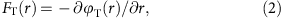where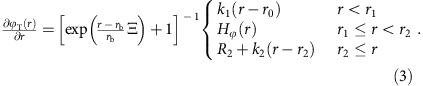In equation (3), ki and ri are spring constants that derived directly from the force-extension curve of the experimental tensile test of the byssus thread at different regions. The Fermi–Dirac distribution function introduces two additional parameters rb and Ξ28. The parameter rb denotes the critical separation distance for breaking of the filament and the parameter Ξ describes the amount of smoothing around the breaking point (the smaller Ξ, the smoother the curve becomes). The parameters R1=Hφ(r=r1)=k1(r1r0) and R2 are calculated from force continuity conditions. Hφ is the Hermite interpolation function that is used to smoothly connect the two linear curves29, which is defined as: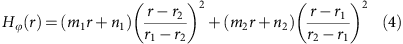Where mi and ni are coefficients determined by the connectivity conditions as

Hφ(r=r1)=R1, Hφ(r=r2)=R2, Hφ(r=r1)=k1 and Hφ(r=r2)=k2 and hence their values are given by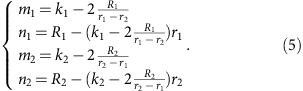An angular spring between two neighbouring springs is used to define the bending stiffness of the thread, and it is given by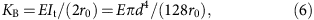where E=4k1r0/(πd2) is the Young’s modules of the thread with different values for the two materials. The bending energy of each angular spring is φB(θ)=KB(θπ)2, where θ is the angle between two neighbouring springs.

Damping effects are included in the model by considering the energy dissipation of each byssus thread in motion caused by the viscosity of water environment. Because of the small dimension of the byssus thread, we use the Stokes’ law to measure the drag force by30: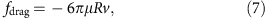where μ=8.6 × 10−4 Pa s is the fluid viscosity constant of water at room temperature and R is the equivalent spherical radius of the mesoscopic bead, which equals to the radius of a sphere of equivalent volume31. The parameter v denotes the relative velocity of particle motion in water. The numerical values of all the parameters for the model are summarized in Supplementary Table S2.

The adhesion plaque is modelled by a rectangular elastic network. The measurement results in a plaque size of l × l=1.9 × 1.9 mm2 as shown in Supplementary Fig. S2. The entire network is composed of n=100 beads, leading to the lattice length as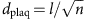. We use the density and Young’s modulus of the distal part to model the stiffness of the adhesive plaque. Considering that the plaque thickness equals to the thread diameter, the mass of each plaque bead is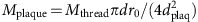and the stiffness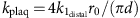. The angular term of the plaque network is determined by the bending stiffness of the network in the two main axis as KB=Ed3/24. The adhesion strength between the plaque and the substrate is modelled according to the single-thread tensile result. For each adhesion point, the strength is given by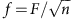, where F=0.4 N is the average strength of a single thread. The adhesion energy can be estimated by γ=nfδ/l2 where δ=30 Å is the detachment distance as has been experimentally measured7. The result yields to 3.3 mJ m−2, which agrees to mussel foot protein interaction strengths on mica measured by the surface forces apparatus10.

### Reaction force on the mussel

The effective stiffness of the network is computed by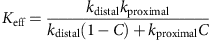where kdistal and kproximal are the unit length stiffness of the distal part and the proximal part. The effective coefficient of viscosity is given by ηeff=ηdistalC+ηproximal(1−C) where ηdistal and ηproximal are the viscosity of the distal part and the proximal part. The reaction force applied to the mussel is thereby expressed as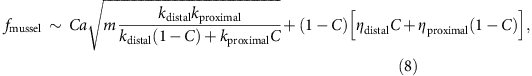where a is a factor that measures the ratio of the contributions of the two forces to the total reaction force on the mussel. It has the physical meaning to measure how an elastic force increment relates to the increment of the total reaction force. We note that this parameter is different from C, which gives the ratio between two portions of a single thread but does not account for the network structure. The stiffness for the two parts is known as we used for modelling as k1 as summarized in Supplementary Table S2 while ηdistal, ηproximal and a are unknown factors in this function. It is noted that ηdistal and ηproximal are not the viscosity of single byssus thread per se, as their values also relate to the structure of the network (R and H here). The value of a also depends on the network structure. For the data points shown in Fig. 6e,f, we test each structure (with fixed R and H) with different C, and take ηdistal, ηproximal and a as parameters and determine their values by fitting. We use this function to fit the simulation results of the normalized reaction force on the mussel and obtain the continuous curve as shown in Fig. 6e,f. The fitting results and the critical C that leads to minimum reaction force are summarized in Supplementary Table S3. The overall value of a is very close to unity, suggesting that both forces are equally important. Moreover, its value increases for larger H/R, indicating that the elastic force becomes slightly more dominating for a narrower and longer network (as its geometry turns from an opened umbrella into a closed one).